# RD Sharma Solutions for Class 6 Maths Chapter 5: Negative Numbers and Integers Objective Type Questions

In the earlier exercises, we understood the problems based on Integers. Here we gain knowledge about the step by step tricks followed to answer the objective type questions which are important from the exam point of view. RD Sharma Solutions for Class 6 prepared by the faculty at BYJU’S consists of answers structured based on the latest CBSE guidelines. To know more about the methods of solving problems, students can refer to RD Sharma Solutions for Class 6 Maths Chapter 5 Negative Numbers and Integers Objective Type Questions PDF present here.

## RD Sharma Solutions for Class 6 Maths Chapter 5: Negative Numbers and Integers Objective Type Questions Download PDF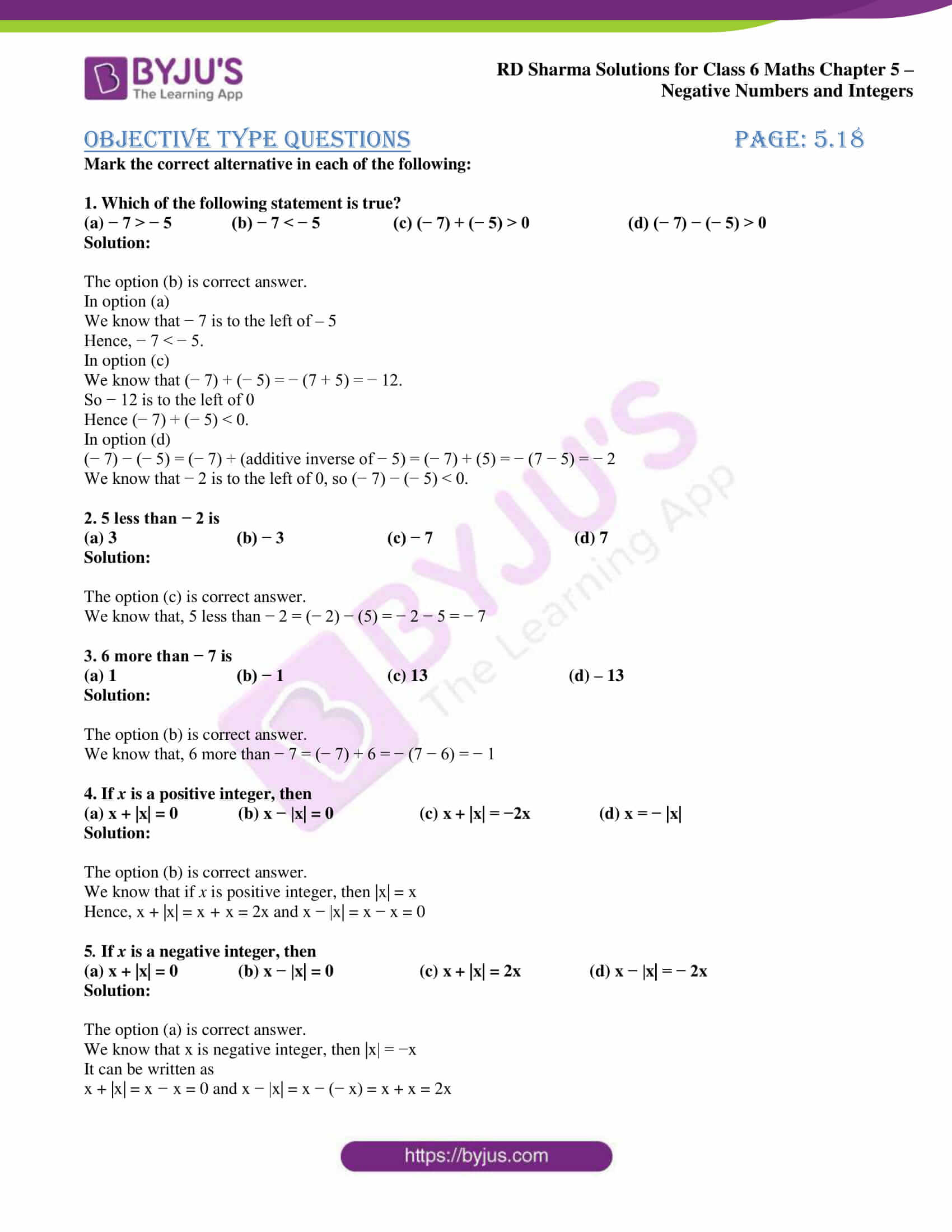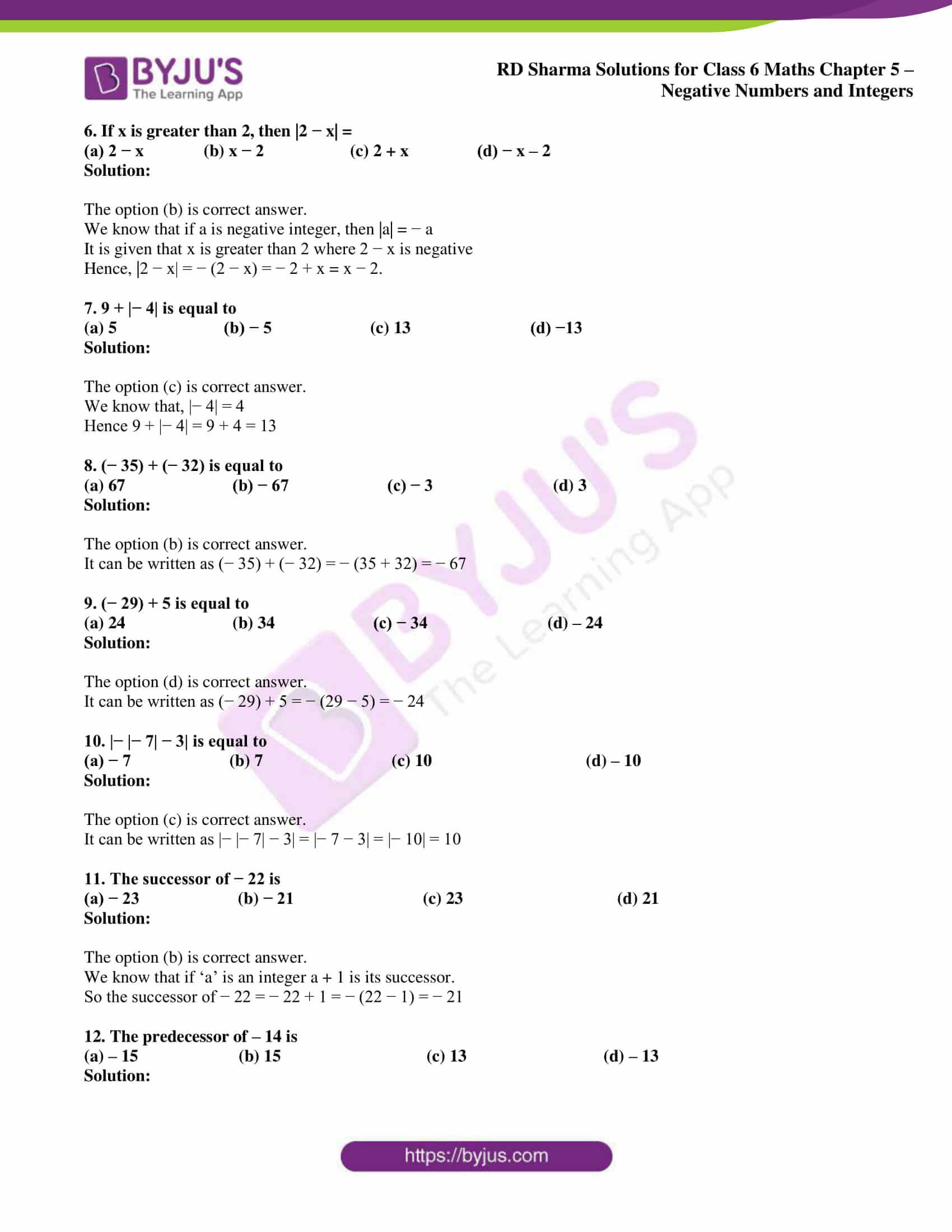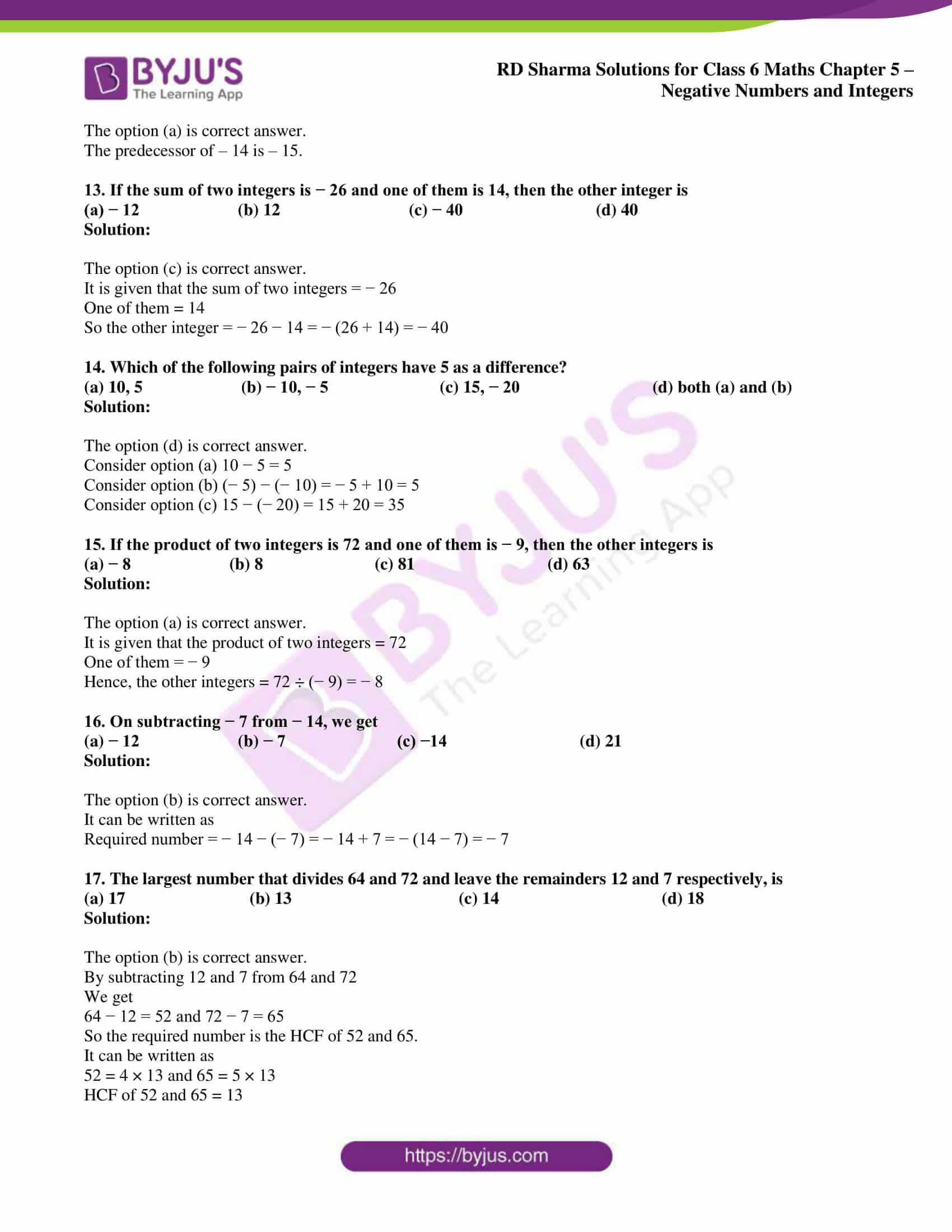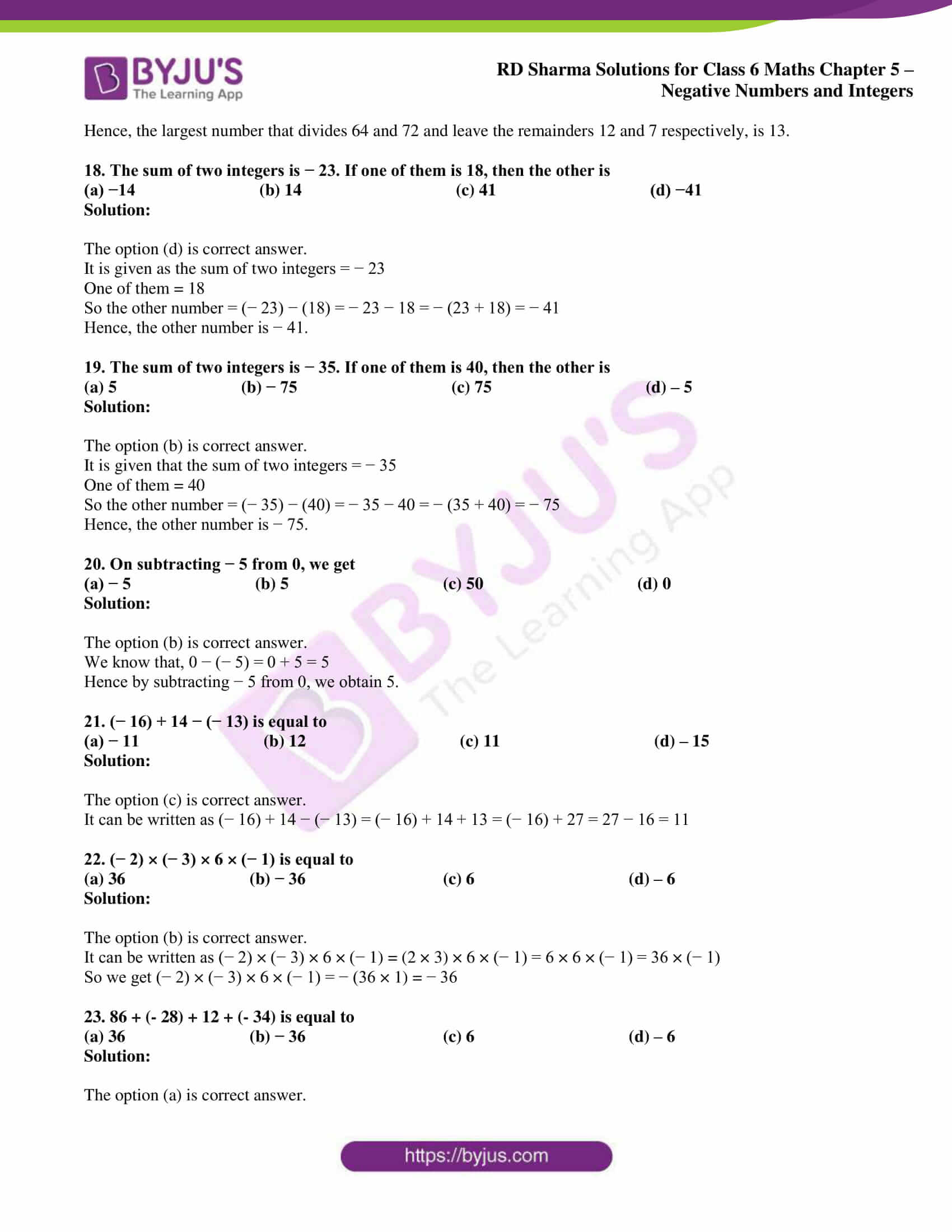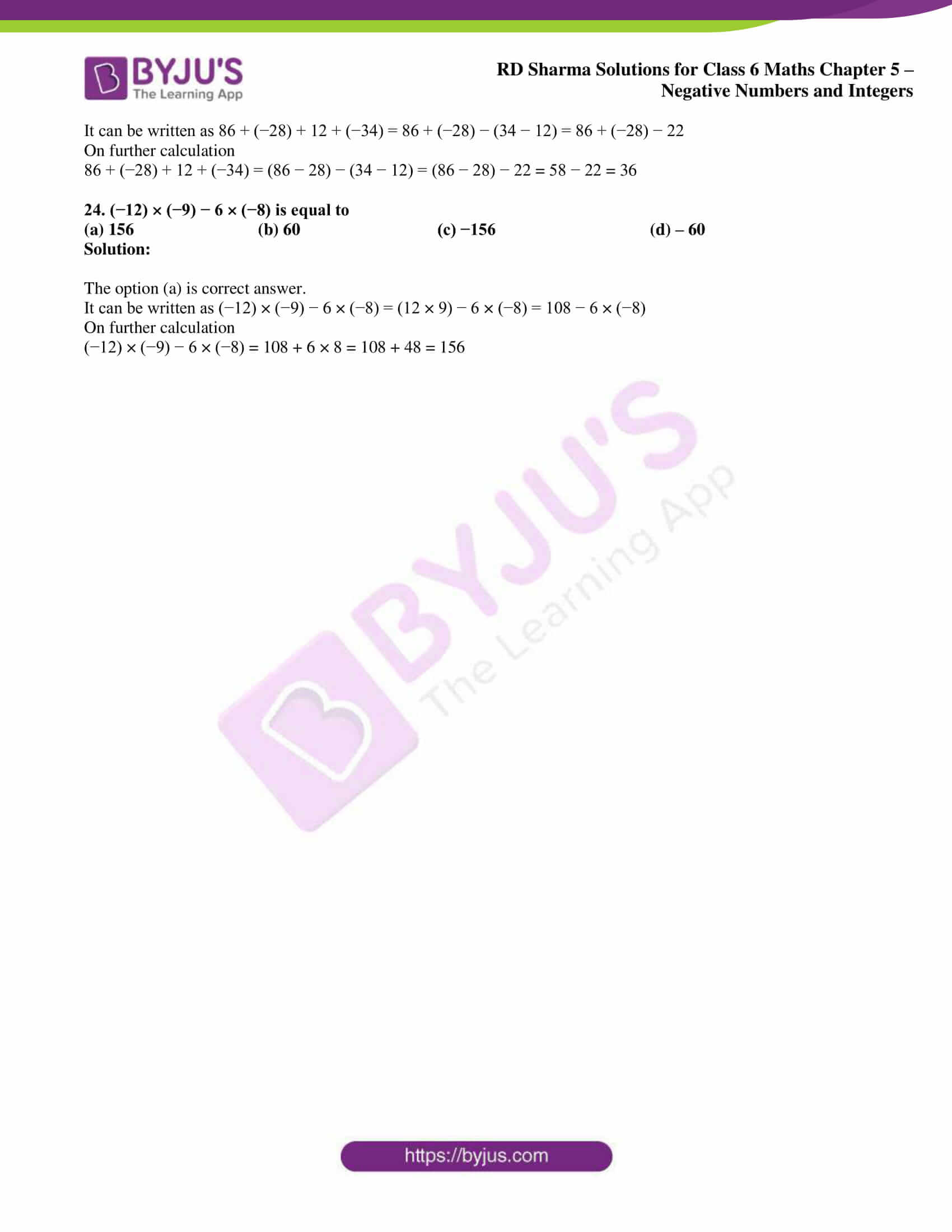### Access answers to Maths RD Sharma Solutions for Class 6 Chapter 5: Negative Numbers and Integers Objective Type Questions

Mark the correct alternative in each of the following:

1. Which of the following statement is true?
(a) − 7 > − 5              (b) − 7 < − 5                 (c) (− 7) + (− 5) > 0                       (d) (− 7) − (− 5) > 0

Solution:

The option (b) is correct answer.

In option (a)

We know that − 7 is to the left of – 5

Hence, − 7 < − 5.

In option (c)

We know that (− 7) + (− 5) = − (7 + 5) = − 12.

So − 12 is to the left of 0

Hence (− 7) + (− 5) < 0.

In option (d)

(− 7) − (− 5) = (− 7) + (additive inverse of − 5) = (− 7) + (5) = − (7 − 5) = − 2

We know that − 2 is to the left of 0, so (− 7) − (− 5) < 0.

2. 5 less than − 2 is
(a) 3                            (b) − 3                        (c) − 7                                 (d) 7

Solution:

The option (c) is correct answer.

We know that, 5 less than − 2 = (− 2) − (5) = − 2 − 5 = − 7

3. 6 more than − 7 is
(a) 1                            (b) − 1                        (c) 13                                 (d) – 13

Solution:

The option (b) is correct answer.

We know that, 6 more than − 7 = (− 7) + 6 = − (7 − 6) = − 1

4. If x is a positive integer, then
(a) x + |x| = 0              (b) x − |x| = 0                    (c) x + |x| = −2x                (d) x = − |x|

Solution:

The option (b) is correct answer.

We know that if x is positive integer, then |x| = x

Hence, x + |x| = x + x = 2x and x − |x| = x − x = 0

5. If x is a negative integer, then
(a) x + |x| = 0              (b) x − |x| = 0                    (c) x + |x| = 2x                (d) x − |x| = − 2x

Solution:

The option (a) is correct answer.

We know that x is negative integer, then |x| = −x

It can be written as

x + |x| = x − x = 0 and x − |x| = x − (− x) = x + x = 2x

6. If x is greater than 2, then |2 − x| =
(a) 2 − x              (b) x − 2                    (c) 2 + x                (d) − x – 2

Solution:

The option (b) is correct answer.

We know that if a is negative integer, then |a| = − a

It is given that x is greater than 2 where 2 − x is negative

Hence, |2 − x| = − (2 − x) = − 2 + x = x − 2.

7. 9 + |− 4| is equal to
(a) 5                         (b) − 5                       (c) 13                            (d) −13

Solution:

The option (c) is correct answer.

We know that, |− 4| = 4

Hence 9 + |− 4| = 9 + 4 = 13

8. (− 35) + (− 32) is equal to
(a) 67                         (b) − 67                       (c) − 3                            (d) 3

Solution:

The option (b) is correct answer.

It can be written as (− 35) + (− 32) = − (35 + 32) = − 67

9. (− 29) + 5 is equal to
(a) 24                         (b) 34                       (c) − 34                            (d) – 24

Solution:

The option (d) is correct answer.

It can be written as (− 29) + 5 = − (29 − 5) = − 24

10. |− |− 7| − 3| is equal to
(a) − 7                       (b) 7                              (c) 10                                    (d) – 10

Solution:

The option (c) is correct answer.

It can be written as |− |− 7| − 3| = |− 7 − 3| = |− 10| = 10

11. The successor of − 22 is
(a) − 23                       (b) − 21                              (c) 23                                    (d) 21

Solution:

The option (b) is correct answer.

We know that if ‘a’ is an integer a + 1 is its successor.

So the successor of − 22 = − 22 + 1 = − (22 − 1) = − 21

12. The predecessor of – 14 is

(a) – 15 (b) 15 (c) 13 (d) – 13

Solution:

The option (a) is correct answer.

The predecessor of – 14 is – 15.

13. If the sum of two integers is − 26 and one of them is 14, then the other integer is
(a) − 12                       (b) 12                              (c) − 40                               (d) 40

Solution:

The option (c) is correct answer.

It is given that the sum of two integers = − 26

One of them = 14

So the other integer = − 26 − 14 = − (26 + 14) = − 40

14. Which of the following pairs of integers have 5 as a difference?
(a) 10, 5                       (b) − 10, − 5                          (c) 15, − 20                               (d) both (a) and (b)

Solution:

The option (d) is correct answer.

Consider option (a) 10 − 5 = 5

Consider option (b) (− 5) − (− 10) = − 5 + 10 = 5

Consider option (c) 15 − (− 20) = 15 + 20 = 35

15. If the product of two integers is 72 and one of them is − 9, then the other integers is
(a) − 8                       (b) 8                          (c) 81                               (d) 63

Solution:

The option (a) is correct answer.

It is given that the product of two integers = 72

One of them = − 9

Hence, the other integers = 72 ÷ (− 9) = − 8

16. On subtracting − 7 from − 14, we get
(a) − 12                       (b) − 7                          (c) −14                               (d) 21

Solution:

The option (b) is correct answer.

It can be written as

Required number = − 14 − (− 7) = − 14 + 7 = − (14 − 7) = − 7

17. The largest number that divides 64 and 72 and leave the remainders 12 and 7 respectively, is
(a) 17                             (b) 13                                       (c) 14                                      (d) 18

Solution:

The option (b) is correct answer.

By subtracting 12 and 7 from 64 and 72

We get

64 − 12 = 52 and 72 − 7 = 65

So the required number is the HCF of 52 and 65.

It can be written as

52 = 4 × 13 and 65 = 5 × 13

HCF of 52 and 65 = 13

Hence, the largest number that divides 64 and 72 and leave the remainders 12 and 7 respectively, is 13.

18. The sum of two integers is − 23. If one of them is 18, then the other is
(a) −14                             (b) 14                                    (c) 41                                    (d) −41

Solution:

The option (d) is correct answer.

It is given as the sum of two integers = − 23

One of them = 18

So the other number = (− 23) − (18) = − 23 − 18 = − (23 + 18) = − 41

Hence, the other number is − 41.

19. The sum of two integers is − 35. If one of them is 40, then the other is
(a) 5                             (b) − 75                                    (c) 75                                    (d) – 5

Solution:

The option (b) is correct answer.

It is given that the sum of two integers = − 35

One of them = 40

So the other number = (− 35) − (40) = − 35 − 40 = − (35 + 40) = − 75

Hence, the other number is − 75.

20. On subtracting − 5 from 0, we get
(a) − 5                             (b) 5                                    (c) 50                                    (d) 0

Solution:

The option (b) is correct answer.

We know that, 0 − (− 5) = 0 + 5 = 5

Hence by subtracting − 5 from 0, we obtain 5.

21. (− 16) + 14 − (− 13) is equal to
(a) − 11                             (b) 12                                    (c) 11                                    (d) – 15

Solution:

The option (c) is correct answer.

It can be written as (− 16) + 14 − (− 13) = (− 16) + 14 + 13 = (− 16) + 27 = 27 − 16 = 11

22. (− 2) × (− 3) × 6 × (− 1) is equal to
(a) 36                             (b) − 36                                (c) 6                                    (d) – 6

Solution:

The option (b) is correct answer.

It can be written as (− 2) × (− 3) × 6 × (− 1) = (2 × 3) × 6 × (− 1) = 6 × 6 × (− 1) = 36 × (− 1)

So we get (− 2) × (− 3) × 6 × (− 1) = − (36 × 1) = − 36

23. 86 + (- 28) + 12 + (- 34) is equal to
(a) 36                             (b) − 36                                (c) 6                                    (d) – 6

Solution:

The option (a) is correct answer.

It can be written as 86 + (−28) + 12 + (−34) = 86 + (−28) − (34 − 12) = 86 + (−28) − 22

On further calculation

86 + (−28) + 12 + (−34) = (86 − 28) − (34 − 12) = (86 − 28) − 22 = 58 − 22 = 36

24. (−12) × (−9) − 6 × (−8) is equal to
(a) 156                             (b) 60                                (c) −156                                    (d) – 60

Solution:

The option (a) is correct answer.

It can be written as (−12) × (−9) − 6 × (−8) = (12 × 9) − 6 × (−8) = 108 − 6 × (−8)

On further calculation

(−12) × (−9) − 6 × (−8) = 108 + 6 × 8 = 108 + 48 = 156